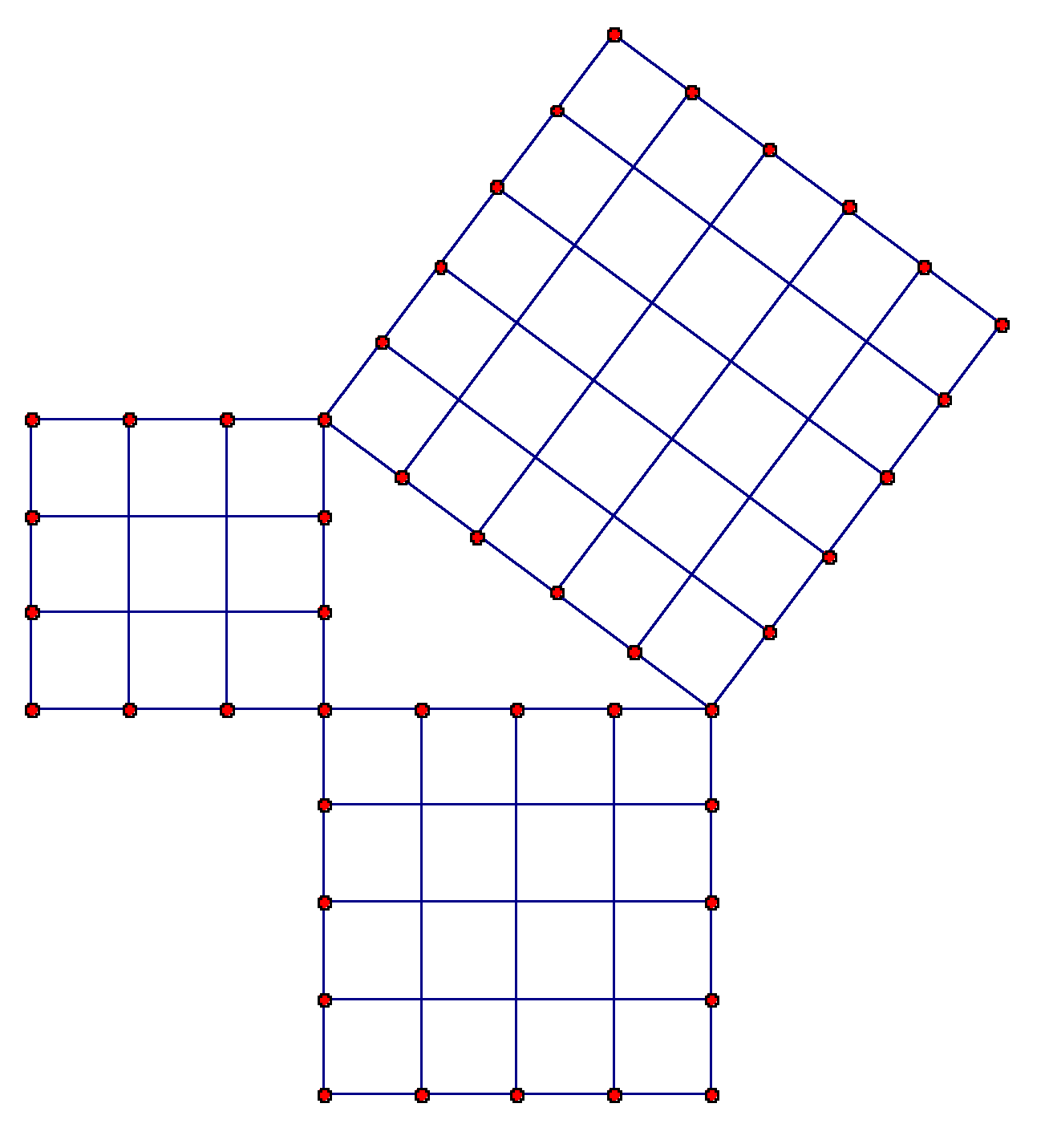# The Night Thread Examines 25

25 is, like all natural numbers1, interesting. Let’s look at a few facts about it.

• It is a square, with prime factorization 5×5. It is also the smallest square equal to the sum of two smaller squares (3²+4²=5²), leading to its frequent use in illustrations of the Pythagorean theorem as seen in the header image.
• It is the sum of the single-digit odd natural numbers (1,3,5,7,9).
• It is one of only two two-digit numbers which always appears at the end of its own higher powers (e.g. 25² = 625), the other being 76.
• It is the smallest base-10 Friedman number (a number which can be computed by applying non-trivial arithmetic operations to its individual digits), being equal to 5².
• It is the number of cents in a US quarter, the smallest coin worth the hassle.
• It is the number of electoral college votes Florida awarded in the 1992, 1996, and (infamously) 2000 US Presidential elections.
• It is the number of extant moving rides at the Magic Kingdom (according to the official lineup of the Parkeology challenge).
• It is the minimum age to join the US House of Representatives, and also to rent a car without paying extra.
• It is the eponymous age of Adele when she created the album 25.
• It is the atomic number of Manganese.
• It is the age I turned today.